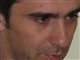# QlikView App Dev

Discussion Board for collaboration related to QlikView App Development.

Announcements
Become an analytics expert with Qlik's new 15 week course: Applied Data Analytics using Qlik Sense. READ MORE
cancel
Showing results for
Did you mean:Not applicable

## Prior year calculation problem

### Hi guys. Currently I have a actual sales calculation like this

=sum(SalesAmount)-sum(SalesAmount*(DiscPrcnt/100))-sum(CreditAmount)+sum(CreditAmount*(CNDiscPrcnt/100))

I want to compare prior year actual sales with current year actual sales. I got this formula in the forums:

num(sum({\$<Year = {\$(=only(Year) - 1)}, Month = {"<=\$(=max(Month))"}>} SalesAmount), '#,##0;(#,##0)')

I do not know how to place the actual sales calculation as mentioned on top into this formula. As you will see that this formula only sum(SalesAmount), but I need to add the whole calc as shown on top.

1 Solution

Accepted SolutionsContributor III

Try like this

=num(

sum({\$<Year = {\$(=only(Year) - 1)}, Month = {"<=\$(=max(Month))"}>} SalesAmount)-

sum({\$<Year = {\$(=only(Year) - 1)}, Month = {"<=\$(=max(Month))"}>} SalesAmount*(DiscPrcnt/100))-

sum({\$<Year = {\$(=only(Year) - 1)}, Month = {"<=\$(=max(Month))"}>} CreditAmount)+

sum({\$<Year = {\$(=only(Year) - 1)}, Month = {"<=\$(=max(Month))"}>} CreditAmount*(CNDiscPrcnt/100))

, '#,##0;(#,##0)')

2 RepliesContributor III

Try like this

=num(

sum({\$<Year = {\$(=only(Year) - 1)}, Month = {"<=\$(=max(Month))"}>} SalesAmount)-

sum({\$<Year = {\$(=only(Year) - 1)}, Month = {"<=\$(=max(Month))"}>} SalesAmount*(DiscPrcnt/100))-

sum({\$<Year = {\$(=only(Year) - 1)}, Month = {"<=\$(=max(Month))"}>} CreditAmount)+

sum({\$<Year = {\$(=only(Year) - 1)}, Month = {"<=\$(=max(Month))"}>} CreditAmount*(CNDiscPrcnt/100))

, '#,##0;(#,##0)')Not applicable
Author
Thank you. This works perfectly.Community Browser